# PSAT Math : How to find the part from the whole

## Example Questions

### Example Question #7 : Whole And Part

If a TV show is 45 minutes long, what fraction of the show is completed 5 minutes after it begins?

Possible Answers:

1/4

1/3

1/10

1/9

1/2

Correct answer:

1/9

Explanation:

5 minutes out of 45 have been completed. We can represent this as a fraction: 5/45. When simplified, this yields 1/9.

### Example Question #8 : Whole And Part

A birthday cake is cut into 8 pieces.  On the first night three pieces are eaten.  On the second night, 20% of the remaining cake was eaten.  On the third night, half of the remaining cake was eaten.  How many slices were left to eat on the fourth night?

Possible Answers:

4.5

3

2

1/2

1

Correct answer:

2

Explanation:

First night: 8 – 3 = 5 remaining.

Second night: 20% of 5 remaining = .2 * 5 = 1 slice Therefore 4 remaining.

Third night: ½ of the 4 remaining = ½*4 = 2 slices remaining

### Example Question #9 : Whole And Part

There was a malfunction at a candy factory and the machines only put red, purple, and green candy into the bags.  If 1/4 of the candies were red and 3/8 were green, how many purple candies were in the bag, if each bag can hold 16 pieces?

Possible Answers:

10

6

8

4

3

Correct answer:

6

Explanation:

Since 1/4 of the 16 are red: 16 * 1/4 = 4 red candies.  With 3/8 being green:

16 X 3/8 = 6 green candies.   16 – 4 – 6 = 6 candies remain, which must be purple.

### Example Question #10 : Whole And Part

In the 30-day month of January, for every three days it snowed, there were seven days it did not snow. The number of days in January on which it did not snow was how much greater than the number of days in January on which it snowed?

Possible Answers:

11

12

14

13

10

Correct answer:

12

Explanation:

The question tells us that for every ten-day period in January (a three-day period plus a seven-day period), it snowed on 3 of those days and did not snow on 7 of those days. Since January has 30 days, it has 3 ten-day periods, so we multiply the numbers given for the 10-day period by 3 to find the  number of days with and without snow during the 30-day period. Doing this, we see that it snowed 3 * 3 = 9 days and did not snow 7 * 3 = 21 days during the 30-day period. Since the question asks how much greater the number of days on which it did not snow is than the number of days on which it snowed, we subtract as follows: number of days it did not snow - number of days it snowed = 21 – 9 = 12.

### Example Question #571 : Arithmetic

Mikey has one full box of cereal.  On Saturday, he eats 1/3 of the the box.  On Sunday he eats 2/3 of what is left.  How much of the box is still left?

Possible Answers:

5/9

2/9

none

4/9

2/3

Correct answer:

2/9

Explanation:

If Mikey eats 1/3 of the box, he is left with 1 – 1/3 = 2/3.

When he eats 2/3 of what remains, he is eating 2/3 of 2/3, or 4/9 of the box.

2/3 – 4/9 = 2/9

### Example Question #572 : Arithmetic

What is the remainder when 27 is divided by 6?

Possible Answers:

0.5

4.5

8

4

3

Correct answer:

3

Explanation:

Long division is the fastest way: 6 goes into 27 four times. 6 times four is 24. 27 – 24 = 3.

### Example Question #11 : Whole And Part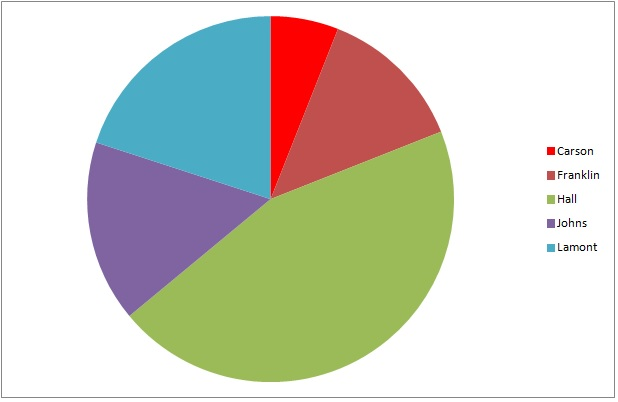The above graph represents the results of a general election for mayor of Kingston.

If 6,239 people voted in the election, which is closest to the number of people who voted for Johns?

Possible Answers: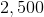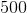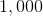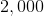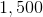Correct answer:Explanation:

The dark purple wedge, which represents Johns, is about one-sixth of the circle, so roughly one-sixth of the voters chose him. This is about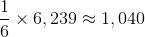so 1,000 is the best choice among the ones given.

### Example Question #12 : Whole And Part

Kim weighs two-thirds as much as Jim. Jim weighs 50 pounds more than Tim. Together, Kim, Jim and Tim weight 430 pounds. How much do Jim and Kim weigh together?

Possible Answers: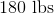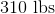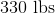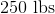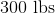Correct answer:Explanation:

Create an equation to represent the combined weights, in pounds, of Kim, Jim and Tim.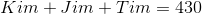Now, taking the other information we are given, put the weights of Kim and Tim in terms of Jim:

Kim weighs two-thirds of Jim: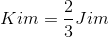Jim weighs 50 pounds more than Tim is the same as saying that Tim weighs 50 pounds less than Jim: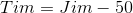Replace the variables of Kim and Jim in the equation with their substitutions: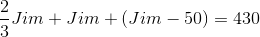Solve for Jim's weight. To combine the fractions, put all variables in terms of thirds and combine like variables: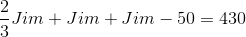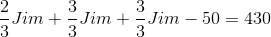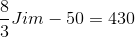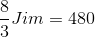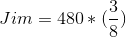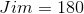Jim weighs 180 pounds. Kim weighs two-thirds of 180: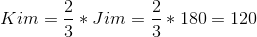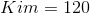The combined weight of Jim and Kim is equal to: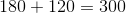### Example Question #13 : Whole And Part

There are 60 ties on a rack in the department store. One-third are blue, two-fifths are green, and the remainder are red. How many red ties are on the rack in the department store?

Possible Answers:

12

14

18

11

16

Correct answer:

16

Explanation:

Begin by finding how many blue and green ties there are in the department store. If one-third of 60 ties are blue, then there are 20 blue ties: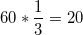Addtionally, if two-fifths of the ties are green, then there are 24 green ties: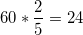To find the number of red ties, subtract the green and blue ties from the total number: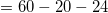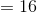There are 16 red ties in the department store.

### Example Question #573 : Arithmetic

A pitcher is currentlyof the way full of water.  Ifounces are poured out, then the pitcher would beof the way full.  How many ounces of water are in the pitcher before theounces are poured out?

Possible Answers: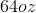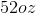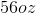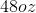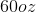Correct answer:Explanation:

Let p represent the total capacity of the pitcher.  The current volume in the pitcher is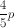.  If this volume is depleted byounces, it becomes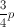.  In other words: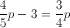Solve this equation for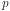and get: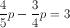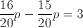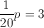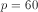Remember,represents the total capacity of the pitcher, or 60 ounces.  The question asks how many ounces are in the pitcher at the beginning of the problem, so evaluate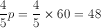.

### All PSAT Math Resources